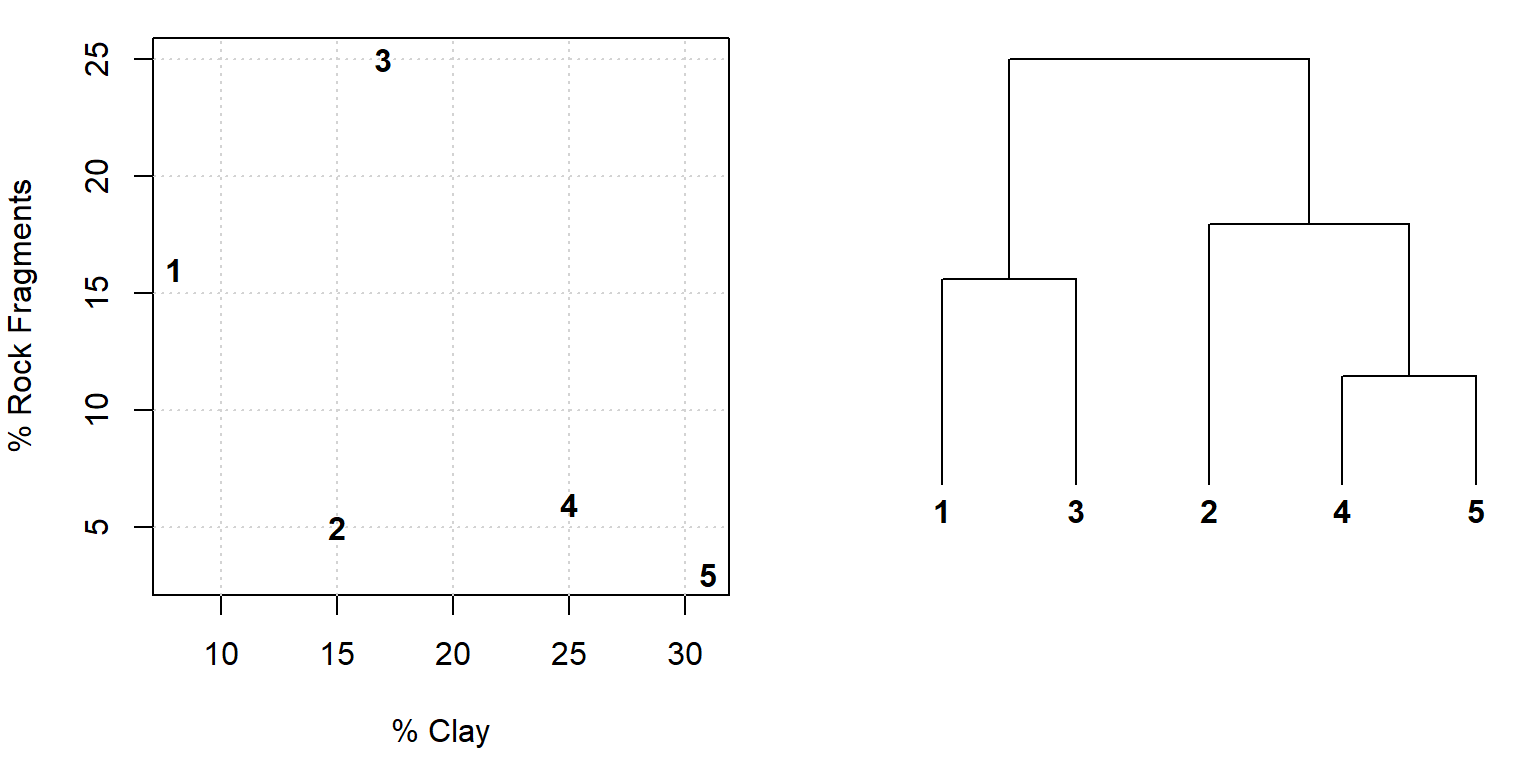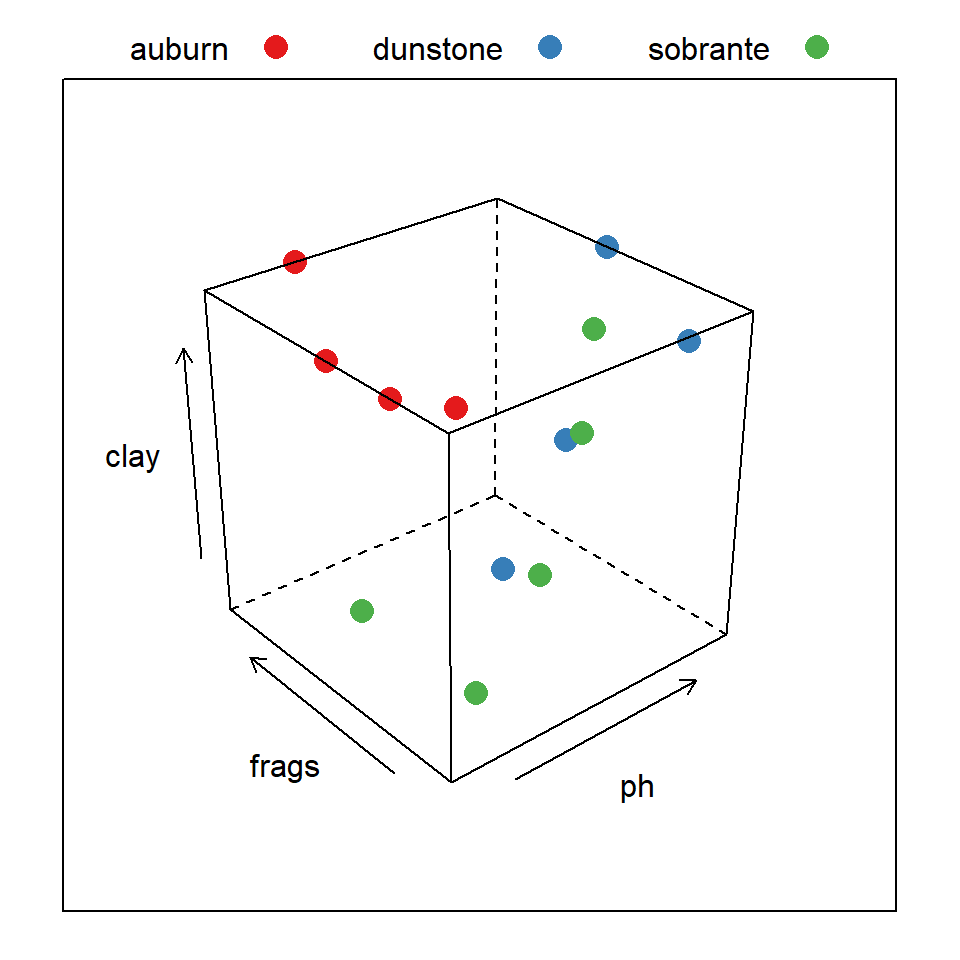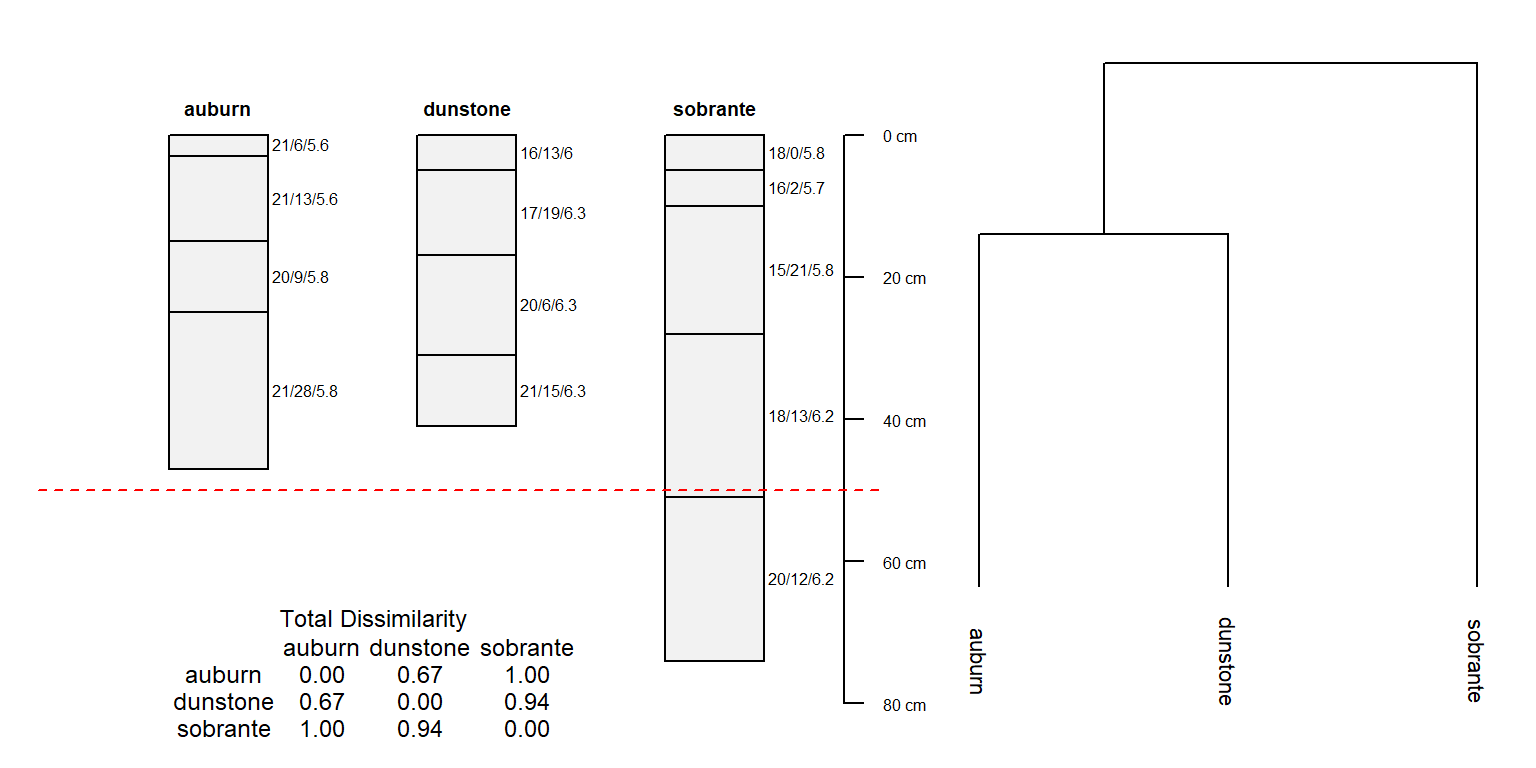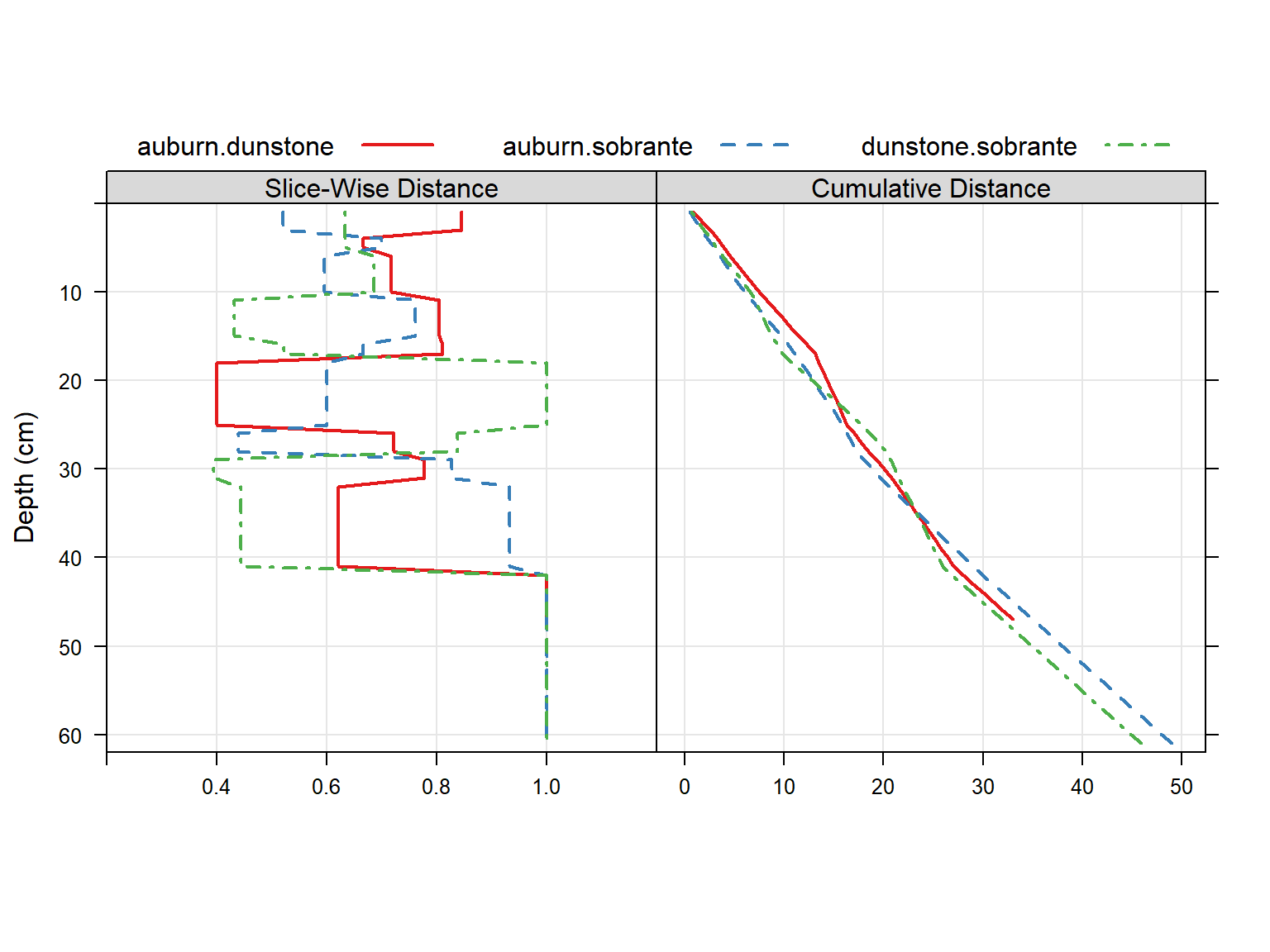# Soil Profile Distances

## Introduction

### Interpreting Dendrograms

Dendrograms are a convenient way of depicting pair-wise dissimilarity between objects, commonly associated with the topic of cluster analysis. This is a complex subject that is best left to experts and textbooks, so I won’t even attempt to cover it here. Unfortunately the interpretation of dendrograms is not very intuitive, especially when the source data are complex. In addition, pair-wise dissimilarity computed between soil profiles and visualized via dendrogram should not be confused with the use of dendrograms in the field of cladistics– where relation to a common ancestor is depicted.

An example is presented below that illustrates the relationship between dendrogram and dissimilarity as evaluated between objects with 2 variables. Essentially, the level at which branches merge (relative to the “root” of the tree) is related to their similarity. In the example below it is clear that (in terms of clay and rock fragment content) soils 4 and 5 are more similar to each other than to soil 2. In addition, soils 1 and 3 are more similar to each other than soils 4 and 5 are to soil 2. Recall that in this case pair-wise dissimilarity is based on the Euclidean distance between soils in terms of their clay content and rock fragment content. Therefore proximity in the scatter plot of frock frags vs. clay is directly related to our simple evaluation of “dissimilarity”. Inline-comments in the code below elaborate further.

# load required libraries
library(cluster) # clustering functions
library(ape) # nicer plotting of dendrograms

# simulate some aggregate profile data
frags <- c(16, 5, 25, 6, 3) # rock fragments
clay <- c(8, 15, 17, 25, 31) # clay contents

# combine into single data.frame
s <- data.frame(clay, frags)

# evaluate pair-wise dissimilarity based on clay and frag %
# since both measurements are on the same scale, no standardization is needed
d <- daisy(s)

# inspect pair-wise dissimilarity matrix
print(d)
## Dissimilarities :
##           1         2         3         4
## 2 13.038405
## 3 12.727922 20.099751
## 4 19.723083 10.049876 20.615528
## 5 26.419690 16.124515 26.076810  6.708204
##
## Metric :  euclidean
## Number of objects : 5
# perform divisive hierarcical clustering for dendrogram creation
d.diana <- diana(d)

# convert object into 'phylo' class for plotting
d.phylo <- as.phylo(as.hclust(d.diana))

# 2-figure plot of original data and resulting dendrogram repsesentation of dissimilarity matrix
par(mfcol=c(1,2), mar=c(4.5,4,1,1))
# original data: setup plot, but don't actually plot it
plot(frags ~ clay, data=s, type='n', xlab='% Clay', ylab='% Rock Fragments')
# add grid lines to assist the eye
grid()
# annotate empty plot with soil numbers
text(s$clay, s$frags, row.names(s), font=2)

# plot dendrogram representation, annotated with the same labels
plot(d.phylo, font=2, label.offset=0.5, adj=0.5, direction='down', srt=90, y.lim=c(-1, 15))### A Soils Example

library(aqp)
library(cluster)
library(ape)
library(RColorBrewer)
library(latticeExtra)
library(plotrix)

# setup some colors
cols <- brewer.pal(3, 'Set1')

# setup horizon-level data: data are from lab sampled pedons
textConnection('
series,top,bottom,clay,frags,ph
auburn,0,3,21,6,5.6
auburn,3,15,21,13,5.6
auburn,15,25,20,9,5.8
auburn,25,47,21,28,5.8
dunstone,0,5,16,13,6
dunstone,5,17,17,19,6.3
dunstone,17,31,20,6,6.3
dunstone,31,41,21,15,6.3
sobrante,0,5,18,0,5.8
sobrante,5,10,16,2,5.7
sobrante,10,28,15,21,5.8
sobrante,28,51,18,13,6.2
sobrante,51,74,20,12,6.2
'), as.is=TRUE)

# establish site-level data
s <- data.frame(
series=c('auburn', 'dunstone', 'sobrante'),
precip=c(24, 30, 32),
stringsAsFactors=FALSE
)

# generate fake horizon names with clay / frags / ph
d\$name <- with(d, paste(clay, frags, ph, sep='/'))

# upgrade to SoilProfile Collection object
depths(d) <- series ~ top + bottom
site(d) <- s

# inspect variables used to determine dissimilarity
cloud(clay ~ ph + frags, groups=series, data=horizons(d),
auto.key=list(columns=3, points=TRUE, lines=FALSE),
par.settings=list(superpose.symbol=list(pch=16, col=cols, cex=1.5))
)# compute betwee-profile dissimilarity, no depth weighting
d.dis <- profile_compare(d, vars=c('clay', 'ph', 'frags'), k=0,

# check total, between-profile dissimilarity, normalized to maximum
d.m <- signif(as.matrix(d.dis / max(d.dis)), 2)
print(d.m)
##          auburn dunstone sobrante
## auburn     0.00     0.67     1.00
## dunstone   0.67     0.00     0.94
## sobrante   1.00     0.94     0.00
# group via divisive hierarchical clustering
d.diana <- diana(d.dis)
# convert classes, for better plotting
d.phylo <- as.phylo(as.hclust(d.diana))

# plot: 2 figures side-by-side
layout(matrix(c(1,2), nrow = 1), widths = c(0.6, 0.4))
par(mar=c(1,1,1,1))
# profiles
plotSPC(d, name='name', axis.line.offset = -1)

# annotate shallow-mod.deep break
abline(h=50, col='red', lty=2)

xjust=0, yjust=0, cex=0.75, title='Total Dissimilarity')

# plot dendrogram in next panel
label.offset=0.5, font=1, y.lim=c(-5, 25), cex=0.7)# continuing from example above...

# return dissimilarity matrices at each depth slice
d.dis.all <- profile_compare(d, vars=c('clay', 'ph', 'frags'), k=0,

# check between-profile dissimilarity, at slice 1
print(as.matrix(d.dis.all[]))
##             auburn  dunstone  sobrante
## auburn   0.0000000 0.8461538 0.5205128
## dunstone 0.8461538 0.0000000 0.6333333
## sobrante 0.5205128 0.6333333 0.0000000
# init functions for extracting pair-wise dissimilarity:
f.12 <- function(i) as.matrix(i)[1, 2] # between auburn and dunstone
f.13 <- function(i) as.matrix(i)[1, 3] # between auburn and sobrante
f.23 <- function(i) as.matrix(i)[2, 3] # between dunstone and sobrante

# apply functions at each slice
d.12 <- sapply(d.dis.all, f.12)
d.13 <- sapply(d.dis.all, f.13)
d.23 <- sapply(d.dis.all, f.23)

# combine into single data.frame
d.all <- make.groups(
auburn.dunstone=data.frame(slice=1:61, d=d.12, d.sum=cumsum(d.12)),
auburn.sobrante=data.frame(slice=1:61, d=d.13, d.sum=cumsum(d.13)),
dunstone.sobrante=data.frame(slice=1:61, d=d.23, d.sum=cumsum(d.23))
)

# plot slice-wise dissimilarity between all three soils
p.1 <- xyplot(slice ~ d, groups=which, data=d.all,
ylim=c(62,0), type=c('l','g'), xlim=c(0.2,1.2), ylab='Depth (cm)', xlab='',
horizontal=TRUE, auto.key=list(columns=3, lines=TRUE, points=FALSE), asp=1)

# plot slice-wise, cumulative dissimilarity between all three soils
p.2 <- xyplot(slice ~ d.sum, groups=which, data=d.all,
ylim=c(62,0), type=c('l','g'), ylab='Depth (cm)', xlab='',
horizontal=TRUE, auto.key=list(columns=3, lines=TRUE, points=FALSE), asp=1)

# combine into panels of a single figure
p.3 <- c('Slice-Wise Distance'=p.1, 'Cumulative Distance'=p.2)
# setup plotting style
tps <- list(superpose.line=list(col=cols, lwd=2, lty=c(1,2,4)), strip.background=list(col=grey(0.85)))
# render figure
print(update(p.3, par.settings=tps))This document is based on aqp version 1.20.